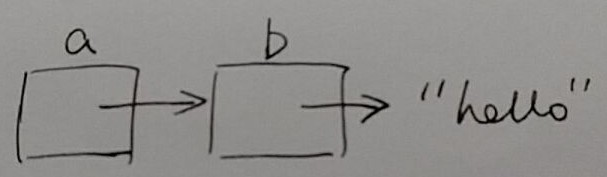# 类似 C 语言中的指针问题, 如何操作?`Emacs Lisp` 学得不好, 不知道如何更好地描述这个问题, 请见谅.

`a` 指向 `"hello"``b` 指向同一个`"hello"`，所以修改 `b` 也就是修改 `a`

``````(let* ((a "hello")
(b a))
(aset b 0 ?H)
a)
;; => "Hello"
``````

`a` 指向 `"hello"``b``car` 指向同一个`“hello”

``````(let* ((a "hello")
(b (list a)))
;; 通过 b 修改 a
(aset (car b) 0 ?H)
a)
;; => "Hello"
``````

（Emacs Lisp 字符串是可修改的，但是实际上一般把字符串当作常量而不修改它）

2 个赞

``````(let ((str '("h" "e" "l" "l" "o"))
(return-str ""))
(setf (car str) "H")
(dolist (el str)
(setq return-str (concat return-str el)))
return-str)

;; ===> "Hello"
``````
1 个赞
``````ELISP> (setq a "hello")
"hello"
ELISP> (setq b 'a)
a
ELISP> (setf (symbol-value b) "world")
ELISP> a
"world"
``````

1 个赞

Emacs Lisp 没法表示「指向一个指针的指针」，所以不会出现你图中的情形。

elisp 没有指针的概念，想操作指针，只能退回到 c 语言层面了，例如楼上几位提到的 aset/set(setf)。

``````#+BEGIN_SRC emacs-lisp :results value
(let* ((a "hello")
(b 'a))
;; (setf (symbol-value b) "world")
;; 等于
;; (set b "world")
;; 等于
(set 'a "world")

;; (aset (symbol-value b) 0 ?W)
;; 等于
(aset a 0 ?W)

a)
#+END_SRC

#+RESULTS:
: World
``````
``````#+BEGIN_SRC C :results output :includes <stdio.h> :flags -std=gnu99 -Wall -Werror
char a = "hello\n";
char *p;

p = &a              // 相当于 (let (... (b 'a)) ...)

sprintf(p, "world\n"); // 相当于 (set b "world")
*p = 'W';              // 相当于 (aset (symbol-value b) 0 ?W)
// a = 'W';         // 相当于 (aset a 0 ?W)

printf("%s\n", a);
#+END_SRC

#+RESULTS:
: World
``````

``````| 操作 | elisp                           | C           |
|------+---------------------------------+-------------|
| 寻址 | (symbol-value b)                | *p          |
| 取址 | 'a                              | &a          |
| 下标 | (aset a 0 ?W)                   | a = 'W'  |
|      | 或 (aset (symbol-value b) 0 ?W) | 或 *p = 'W' |
``````
2 个赞

`(gv-ref)` 相当于 C 的 `&``(gv-deref)` 相当于 C 的 `*`

``````(setq test 'test)

(defun test1 (a)
(setf (gv-deref a) nil))

(test1 (gv-ref test))
test
;; ===> nil
``````
4 个赞